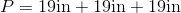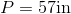# ISEE Upper Level Math : How to find the perimeter of an equilateral triangle

## Example Questions

### Example Question #1 : How To Find The Perimeter Of An Equilateral Triangle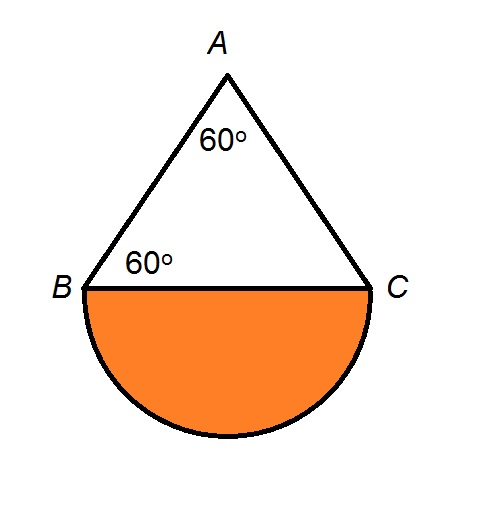Refer to the above figure. The shaded region is a semicircle with area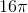. Give the perimeter of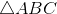.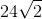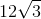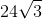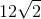Explanation:

Given the radiusof a semicircle, its area can be calculated using the formula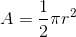.

Substituting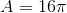: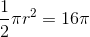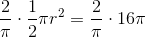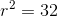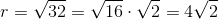The diameter of this semicircle is twice this, which is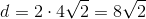; this is also the length of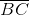.has two angles of degree measure 60; its third angle must also have measure 60, makingan equilateral triangle with sidelength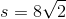. Its perimeter is three times this, or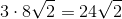### Example Question #2 : How To Find The Perimeter Of An Equilateral Triangle

Find the perimeter of an equilateral triangle with a base of length 8cm.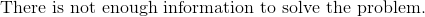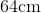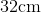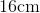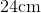Explanation:

To find the perimeter of a triangle, we will use the following formula: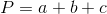where a, b, and c are the lengths of the sides of the triangle

Now, we know the base of the triangle is 8cm.  Because it is an equilateral triangle, all sides are equal.  Therefore, all sides are 8cm.

Knowing this, we can substitute into the formula.  We get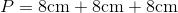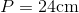### Example Question #3 : How To Find The Perimeter Of An Equilateral Triangle

Find the perimeter of an equilateral triangle with a base of 21in.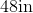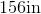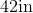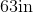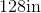Explanation:

To find the perimeter of a triangle, we will use the following formula:where a, b, and c are the lengths of the sides of the triangle.

Now, we know the base of the triangle has a length of 21in.  Because it is an equilateral triangle, all lengths are the same.  Therefore, all lengths are 21in.

Knowing this, we can substitute into the formula.  We get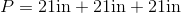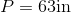### Example Question #4 : How To Find The Perimeter Of An Equilateral Triangle

Find the perimeter of an equilateral triangle with a base of 23in.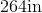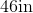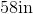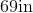Explanation:

To find the perimeter of a triangle, we will use the following formula:where a, b, and c are the lengths of the sides of the triangle.

Now, we know the base of the triangle has a length of 23in.  Because it is an equilateral triangle, all lengths are the same.  Therefore, all lengths are 23in.

Knowing this, we can substitute into the formula.  We get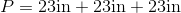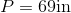### Example Question #5 : How To Find The Perimeter Of An Equilateral Triangle

Find the perimeter of an equilateral triangle with a base of 22in.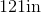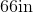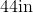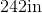Explanation:

An equilateral triangle has 3 equal sides.  So, we will use the following formula: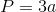where is the length of one side of the triangle.

Now, we know the base of the triangle is 22in.  Because all sides are equal, all sides are 22in.  So, we can substitute into the formula: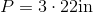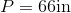### Example Question #6 : How To Find The Perimeter Of An Equilateral Triangle

Find the perimeter of an equilateral triangle with a base of 14in.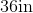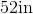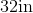Explanation:

An equilateral triangle has 3 equal sides.  To find the perimeter of an equilateral triangle, we will use the following formula:where a is the length of one side of the triangle.

Now, we know the base of the equilateral triangle is 14in. So, we get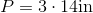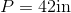### Example Question #7 : How To Find The Perimeter Of An Equilateral Triangle

Find the perimeter of an equilateral triangle with a base of 19in.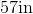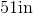Explanation:

To find the perimeter of a triangle, we will use the following formula:where a, b, and c are the lengths of the sides of the triangle.

Now, we know the base of the triangle is 19in. Because it is an equilateral triangle, all sides are equal. Therefore, all sides are 19in. So, we get